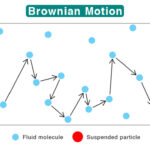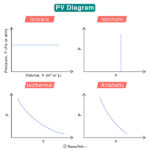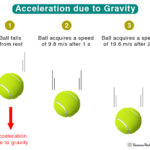Home / Physics / Stefan-Boltzmann Law

# Stefan-Boltzmann Law

The Stefan-Boltzmann law states that “the total radiant power emitted by a surface across all wavelengths is proportional to the fourth power of its absolute temperature”. The law generally applies to black bodies that absorb all radiation falling on them.

Stefan-Boltzman law is named after Austrian physicists Josef Stefan and Ludwig Boltzmann. Stefan formulated the law in 1877, and Boltzmann derived it in 1884.

## Formula

Suppose P is the radiant heat energy emitted per second, A is the surface area of the emitter, and T is the temperature of the surface in absolute scale. According to Stefan-Boltzmann law,

$\frac{P}{A} = \sigma T^4$

Where s is called the Stefan-Boltzmann constant. It has the following expression:

$\sigma = \frac{2p^{5}k_{B}^{4}}{15h^{3}c^{2}}$

Where

kB: Boltzmann’s constant

c: Speed of light

Plugging in the values of kB, h, and c, the Stefan-Boltzmann constant comes out to be 5.67 x 10-8 W · m-2 ·K-4.

For objects that are not black bodies, the Stefan-Boltzmann equation modifies to

$\frac{P}{A} = e\sigma T^4$

Where e is the emissivity of the object. It takes fractional values between 0 and 1. It is 1 for a black body. Therefore, the radiation emitted from an object that is not a black body is less than that emitted by a black body at the same temperature.

Suppose the object radiates energy to its cooler surrounding, which is at a temperature TC. The radiation equation modifies to

$\frac{P}{A} = \sigma (T^4 – T_{C}^4)$

From the above equations, it is clear that the total radiative power increases as the temperature increases, making heated objects glow bright.

Stefan-Boltzmann’s law can be derived using Planck’s radiation formula. Planck’s equation is integrated over wavelength to get the radiative power.Brownian MotionPV DiagramVelocity vs AccelerationAcceleration due to Gravity